Author: Oscar Cronquist Article last updated on January 28, 2020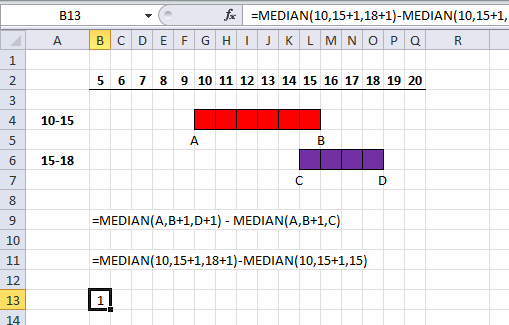This article demonstrates how to calculate overlapping numerical ranges. What is interesting to know is the fact that Excel handles dates as numbers.

This makes it possible to use the methods shown in this article with Excel date and time values. An Excel date is really only an integer (whole number) formatted as a date. For example, 1/1/1900 is 1. 1/1/2000 is 36526.

There are 36525 days between 1/1/1900 and 1/1/2000, you can verify this yourself. Type 1/1/2000 in a cell, select the cell again. Press CTRL + 1 to open the "Format cells" dialog box. Press with left mouse button on "General"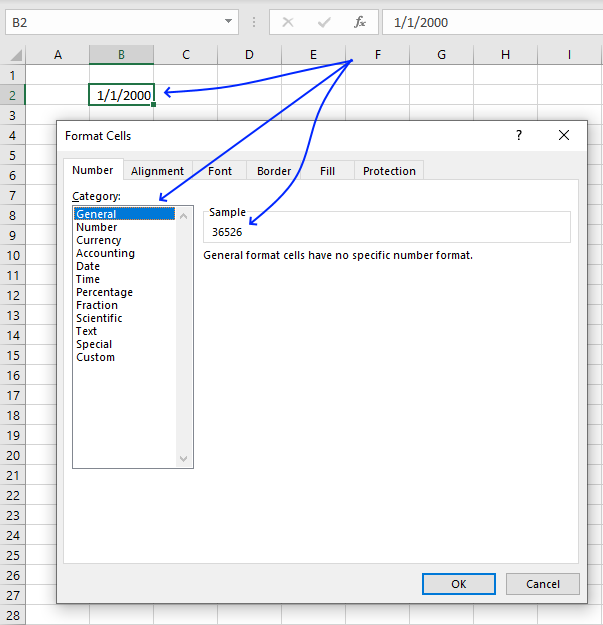Now we know how Excel handles dates. This makes it possible to use the MEDIAN function to calculate the number of overlapping days between two date ranges.

I have five examples below that demonstrate the formula calculations in great detail, one is added to the end number of both ranges and the reason is that date ranges count the end date contrary to numerical ranges.

For example, numerical range 5 to 10 is 10 - 5 equals 5. However, date range 1/5/2020 to 1/10/2020 contains 6 days. That is the reason the formulas below are adding 1 to the end dates of both date ranges.

Hi Oscar,
I need a formula that gives me the number of days contained in a range that overlaps another range.

I got this from Carol Weideman, thank you! I think it is really simple and clever.

Date range 1: A to B and date range 2: C to D

Formula:

=MEDIAN(A,B+1,D+1)-MEDIAN(A,B+1,C)

MEDIAN(number1, [number2], ..)
Returns the median, or the number in the middle of the set of given numbers

The MEDIAN function returns the middle number based on a set of given numbers, the formula above uses two MEDIAN functions and each function contains three numbers.

The first MEDIAN function contains the start date, end date + 1 of the first date range and the end date + 1 of the second date range. The second MEDIAN function contains the start date, end date + 1 of the first date range and the start date of the second date range.

### Example 1 - No overlapping ranges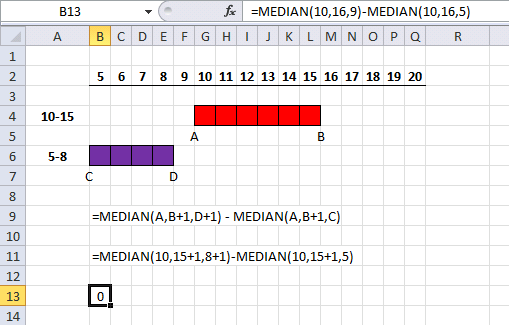This example has two ranges that don't overlap, this demonstrates the calculations behind the formula when it returns 0 (zero) overlapping units.

The first range begins at 10 and ends at 15, the second range begins 5 and ends at 8. The second range is located before the first range, example 5 demonstrates when the second range is located after the first range.

=MEDIAN(A,B+1,D+1) - MEDIAN(A,B+1,C)

becomes

=MEDIAN(10,15+1,8+1)-MEDIAN(10,15+1,5)

becomes

=MEDIAN(10,16,9)-MEDIAN(10,16,5)

becomes

10 - 10 = 0

0 (zero) units are overlapping.

### Example 2 - Partially overlapping ranges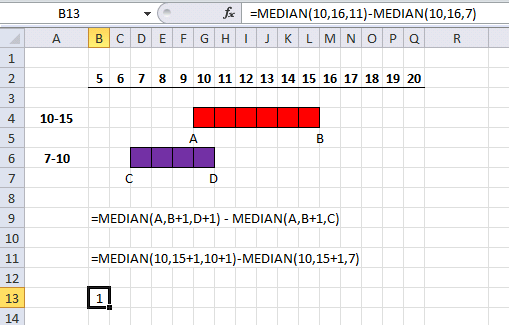This example has two numerical ranges that partially overlap, see image above. The first range begins at 10 and ends at 15.

The second range begins at 7 and ends at 10, the ranges overlap by one unit. Example 4 shows the same setup except that the second range overlaps the last unit of the first range.

=MEDIAN(A,B+1,D+1) - MEDIAN(A,B+1,C)

becomes

=MEDIAN(10,15+1,10+1)-MEDIAN(10,15+1,7)

becomes

=MEDIAN(10,16,11)-MEDIAN(10,16,7)

becomes

11 - 10 = 1

1 unit is overlapping.

### Example 3 - Overlapping ranges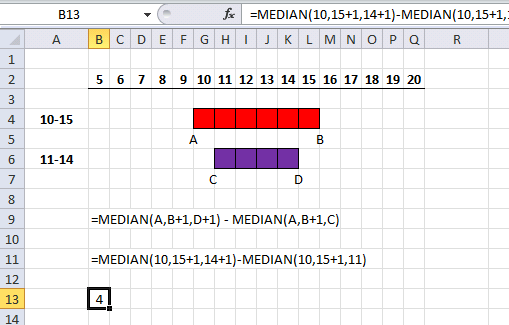This example demonstrates when two ranges completely overlap each other, the first range starts at 10 and ends at 15.

The second range starts at 11 and ends at 14, four units overlap in total which the image above shows.

=MEDIAN(A,B+1,D+1) - MEDIAN(A,B+1,C)

becomes

=MEDIAN(10,15+1,14+1)-MEDIAN(10,15+1,11)

becomes

=MEDIAN(10,16,15)-MEDIAN(10,16,11)

becomes

15 - 11 = 4

4 units are overlapping.

### Example 4 - Partially overlapping rangesThis example shows two ranges that partially overlap, the first range starts at 10 and ends at 15. The second range starts at 15 and ends at 18.

Both ranges overlap at 15 and only one unit is overlapping, see image above.

=MEDIAN(A,B+1,D+1) - MEDIAN(A,B+1,C)

becomes

=MEDIAN(10,15+1,18+1)-MEDIAN(10,15+1,15)

becomes

=MEDIAN(10,16,19)-MEDIAN(10,16,15)

becomes

16 - 15 = 1

1 unit is overlapping

### Example 5 - No overlapping ranges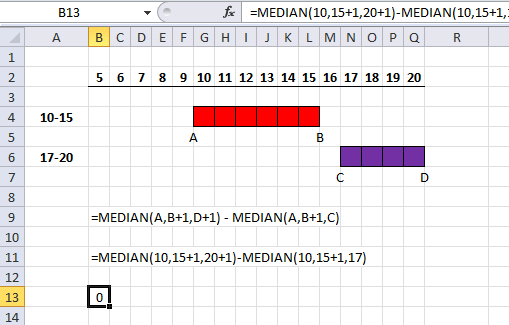The last example

=MEDIAN(A,B+1,D+1) - MEDIAN(A,B+1,C)

becomes

=MEDIAN(10,15+1,20+1)-MEDIAN(10,15+1,17)

becomes

=MEDIAN(10,16,21)-MEDIAN(10,16,17)

becomes

16 - 16 = 0

0 units are overlapping.

### Can these calculations be done with multiple date ranges?

Yes, they can. However, the MEDIAN function is not suitable for array calculations so the formulas are somewhat more complicated.

I have written an article that demonstrates a formula that tells you if a date range is overlapping any other date range specified in a list. It returns True if it is overlapping and False if not.

What if you want to know exactly which date range overlaps?
Check out this article: Identify rows of overlapping records The formula returns the row number or rows of all date ranges that overlap.

Can I use the MEDIAN function to calculate time ranges?
Yes, you can. I have written this article that shows the formula calculations in detail:
How to calculate overlapping time ranges

Check out the Overlapping category to find many more examples and tutorials.

### Get the Excel fileDays-contained-in-a-range-that-overlap-another-range.xlsx